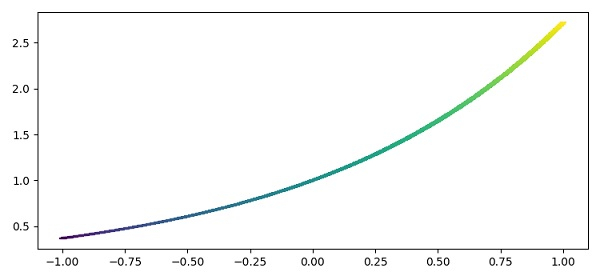# How to plot a gradient color line in matplotlib?

To plot a gradient color line in matplotlib, we can take the following steps −

• Create x, y and c data points, using numpy.

• Create scatter points over the axes (closely so as to get a line), using the scatter() method with c and marker='_'.

• To display the figure, use the show() method.

## Example

import numpy as np
from matplotlib import pyplot as plt
plt.rcParams["figure.figsize"] = [7.50, 3.50]
plt.rcParams["figure.autolayout"] = True
x = np.linspace(-1, 1, 1000)
y = np.exp(x)
c = np.tan(x)
plt.scatter(x, y, c=c, marker='_')
plt.show()

## Output